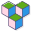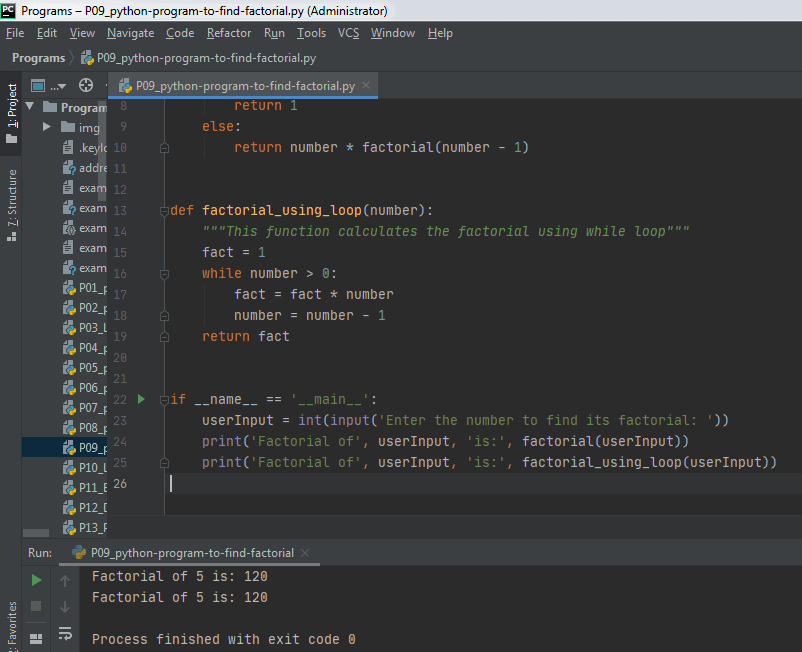CppBuzz# Python program to find factorial

# Author: CppBuzz.com
# This program finds factorial of any number
# Date: 22nd Aug 2020

```
def factorial(number):
"""This function finds the factorial of the number passed as argument"""
if number < 0:
print('Invalid entry! Cannot find factorial of a negative number')
if number == 0 or number == 1:
return 1
else:
return number * factorial(number - 1)

if __name__ == '__main__':
number = int(input('Enter any number8: '))
print(factorial(number))

```